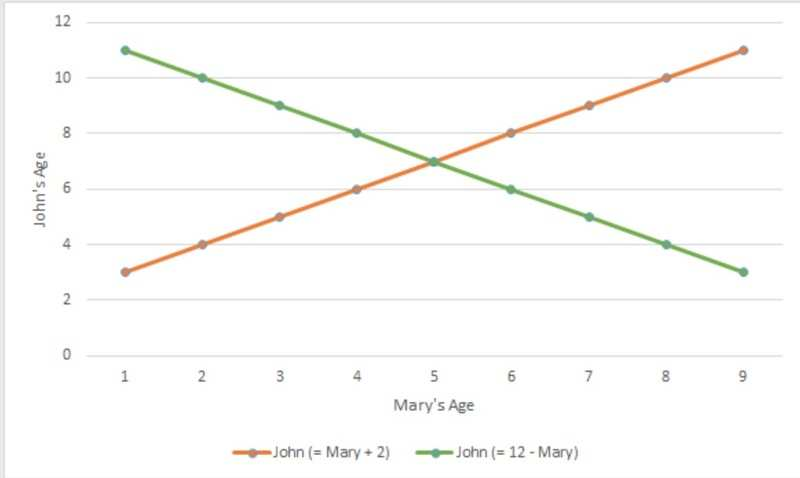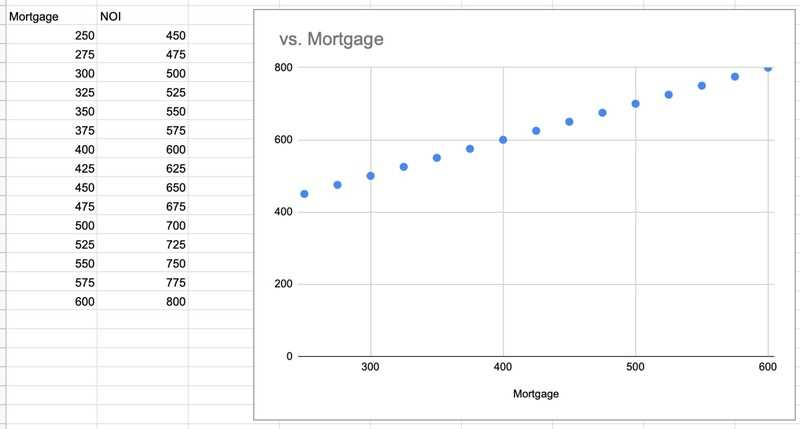Remember math puzzles like this?

John is two years older than Mary, and their ages added together equal 12. How old are they now?

Turns out this can be solved very easily using a graph and two lines. John's age is the Y-axis and Mary's is the X-axis. first you plot John's age as 2 years older than Mary's age, then you plot John's age as 12-Mary's age. The intersection of the two lines is their ages.(I got this from https://www.skillsyouneed.com/num/graphs-charts.html

This got me to think...

Could I use a cartesian graph to find the maximum price I should pay for a house?  i.e. Could I make a graph where the intersection of its lines are \$200/month cashflow?

This is where I need your help...

I have plotted a similar graph using monthly mortgage payments and monthly NOI. I want to know how you would setup your graph to calculate the ideal purchase price and rent rate. What would your X-axis be? Y-Axis? What lines would you plot?

My graph of monthly mortgage payment and monthly NOI turned out to be a single line because I just needed a simple relationship of  NOI - Mortgage = 200.  What else is possible?